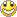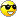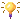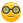Some new stuff
1. Arduino Park Control
2. Arduino USB2serial programming the bare bone
3. Arduino Projects Videos added
4. Arduino Xbee projects
5. Arduino dB Meter
6. Trying to get the IOIO going.
7. IOIO is going....bluetooth8. Attiny 2313 bluetooth bee RC car
9. Compass and temperature added
10. AVR programmer
11. USB2Serial and Ethernet POE
12. Arduino LPG Sensor
13. Program the Arduino Mini 05
14. Lipo Rider Pro in action
15. EZ Robot Builder kit arrived 10 Nov 2012
16. Arduino VA Meter!
17. 05 Nov 2012 Project Sentry Gun.
18. Attiny85 PIR Sensor 10 Dec 2012
19. 0-30VDC3A and 2-28VDC10A bench PSU's20. 19 Dec 2012 Arduino Voltmeter LCD
21. 29 Dec 2012 Arduino Tachometer&Speedometer LCD
22. 03 Jan 2013 Arduino AC Phase Control.
23. 18 Jan 2013 Arduino Distance Meter
24. 22 Jan 2013 Arduino Digital Clock and Date
25. 31 Jan 2013 Arduino Digital Clock and Date 8x2 LCD
26. Feb 2013 LCD and Backpack
27. Feb 2013 7 Segment Serial Clock Sparkfun. Distance Sensor with "newping" library
28. Feb 2013 Lelo Remote
29. 01/03/2013 Arduino Adafruit 7 Seg Digital Clock with RTC and backpack
30. Arduino Frequency Meter 26/03/2013
31. Another RC  Car controlled by Arduino 06/04/2013
32. My quadcopter project update 25 April 2013
33. My Aqua Quad Copter Flying 26 April 201334. Another monster RC truck;strong one! 11July201335. X-Frame Quad Copter 31 July 201336. Arduino GSM Sheild added.28/8/2013
37. 1.8inch TFT screen 13/09/201338. 04/11/2014 added PID Soldering Iron Control
39. Added bluetooth servo control 04/11/2014
40. ESP8266 first try.16/03/2015
41. Arduino Uno Quadcopter 19 May 2015
42. IOT WemosD1 Amp Meter29/03/2013

Arduino Frequency Counter from fizix.com which I slightly altered to display on Adafruit backpack LCD

Code:

// This code measure the frequency of an incoming
// square-wave signal using Timer/Counter 1 and the onboard
// comparator. The signal I am measuring has a duty-cycle of
// 50% but the code should work regardless of the duty-cycle.
// It should also work for non-square-wave signals so long as
// the signal voltage drops below the bandgap voltage of the
// onboard comparator (approximately 1.1 V) and is sufficiently
// clean to prevent bouncing.

// A variable to keep track of the number of overflows on
// Timer/Counter 1.
volatile unsigned int overflows = 0;
#include "Wire.h"
#include "LiquidCrystal.h"
// A4 = SDA,A5 = SCL
LiquidCrystal lcd(0);

// A variable to keep track of how many rising edges of the
// signal have been counted.
volatile unsigned long edges = 0;

// A variable to keep track of the count on Timer/Counter 1
// when I start counting the edges of the signal.
volatile unsigned long tstart = 0;

// A variable to keep track of the count on Timer/Counter 1
// when I stop counting the edges of the signal.
volatile unsigned long tstop = 0;

// A variable to store temporarily store the count on
// Timer/Counter 1.
volatile unsigned long tnow = 0;

// This specifies how many cycles over which I want to
// average the frequency.
const unsigned long cycles = 1000;

// A variable to store the currently measured frequency
float frequency = 0;

void setup(void) {
pinMode(7,INPUT); // This is the analog comparator negative input.
// This is where the input signal enters the Arduino.

SREG = SREG | B10000000; // Enable gobal interrupts. They should
// already be enabled but I like to do this out of good measure.

Serial.begin(9600); // For printing the frequency to the terminal
lcd.begin(16, 2);
}

void loop(void) {
delay(500);
measureFreq();
Serial.println(frequency);
lcd.setCursor(3,0);
lcd.print(frequency);
lcd.setCursor(8,1);
lcd.print("Hz");
delay(500);
}

void measureFreq(void) {
edges = 0;

ACSR = ACSR | B01000010; // enable analog comparator interrupt
// on failing edge (bit 1) which would actually capture a rising
// edge of the signal and use the internal bandgap reference
// voltage as the positive input (bit 6).
delay(5); // A short wait for bandgap voltage to stabilize.

overflows = 0;

TCCR1A = B00000000; // Set Timer/Counter 1 in normal mode where
// it will count to 0xFFFF then repeat.
TIMSK1 = TIMSK1 | B00000001; // Turn on Timer/Counter 1 overflow
// interrupt (bit 0).

// Turn on the counter with no prescaler.
TCCR1B = TCCR1B | B00000001;

ACSR = ACSR | B00001000; // Enable analog comparator interrupt
// (bit 3).

while (edges < (cycles+1)) {
// Do nothing.
}

// Calculate the frequency.
frequency = (float)16000000*(float)cycles/(float)(tstop - tstart);

}

ISR(TIMER1_OVF_vect)
{
overflows += 1;
}

ISR(ANALOG_COMP_vect)
{
tnow = TCNT1; // current time
edges += 1;
if (edges == 1) { // Start counting edges.
tstart = overflows*65536 + tnow;
}
else if (edges == cycles + 1) { // Stop counting edges.
tstop = overflows*65536 + tnow;
// Turn off Timer/Counter 1 and the comparator.
ACSR = 0;
TCCR1B = 0;
}
}